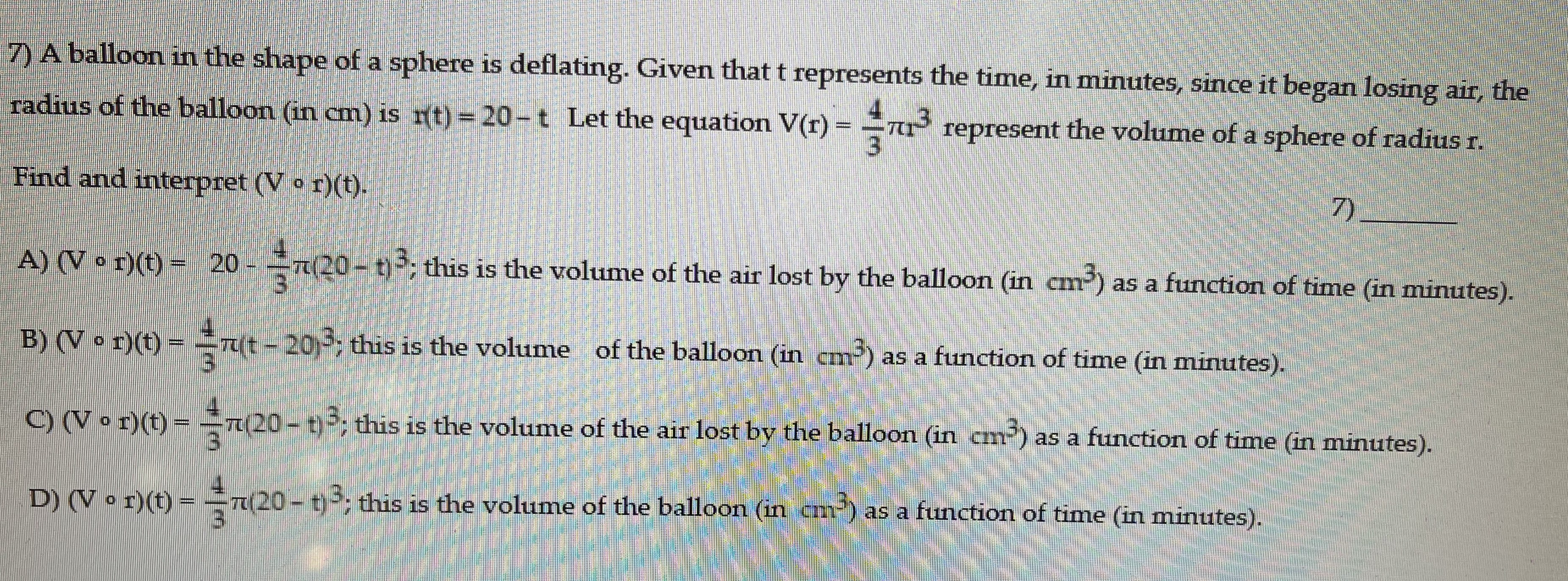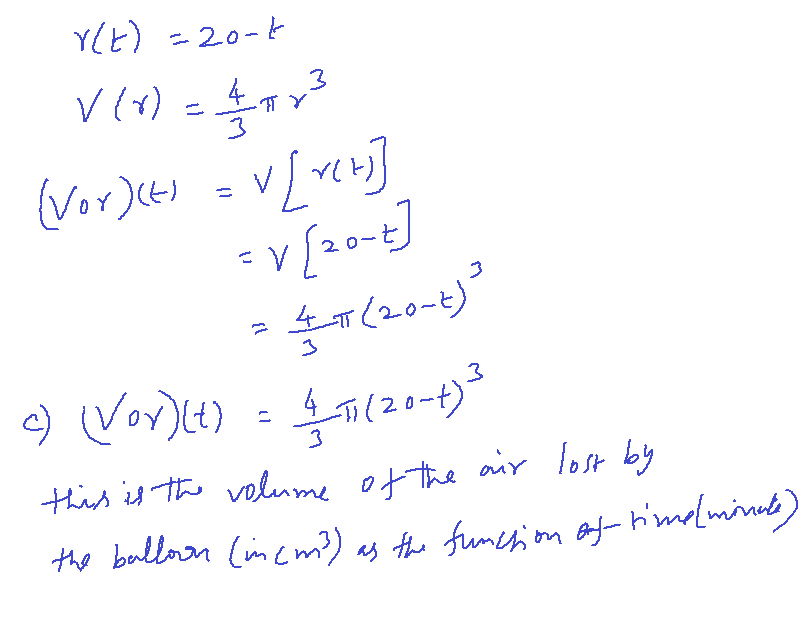### ¿Todavía tienes preguntas de matemáticas?

Pregunte a nuestros tutores expertos
Algebra
Pregunta7) A balloon in the shape of a sphere is deflating. Given that $$t$$ represents the time, in minutes, since it began losing air, the radius of the balloon (in $$cm )$$ is $$r ( t ) = 20 - t$$ Let the equation $$V ( r ) = \frac { 4 } { 3 } \pi r ^ { 3 }$$ represent the volume of a sphere of radius $$r$$ . Find and interpret $$( V \circ r ) ( t )$$ .

A) $$( V \circ r ) ( t ) = 20 - \frac { 4 } { 3 } \pi ( 20 - t ) ^ { 3 }$$ ; this is the volume of the air lost by the balloon (in cm $${ } ^ { 3 }$$ ) as a function of time (in minutes).

B) $$( V \circ r ) ( t ) = \frac { 4 } { 3 } \pi ( t - 20 j ^ { 3 }$$ ; this is the volume of the balloon (in cm) as a function of time (in minutes).

C) $$( V \circ r ) ( t ) = \frac { 4 } { 3 } \pi ( 20 - t ) ^ { 3 }$$ ; this is the volume of the air lost by the balloon (in cm) as a function of time (in minutes).

D) $$( V \circ r ) ( t ) = \frac { 4 } { 3 } \pi ( 20 - t ) ^ { 3 }$$ ; this is the volume of the balloon (in cm³) as a function of time (in minutes).